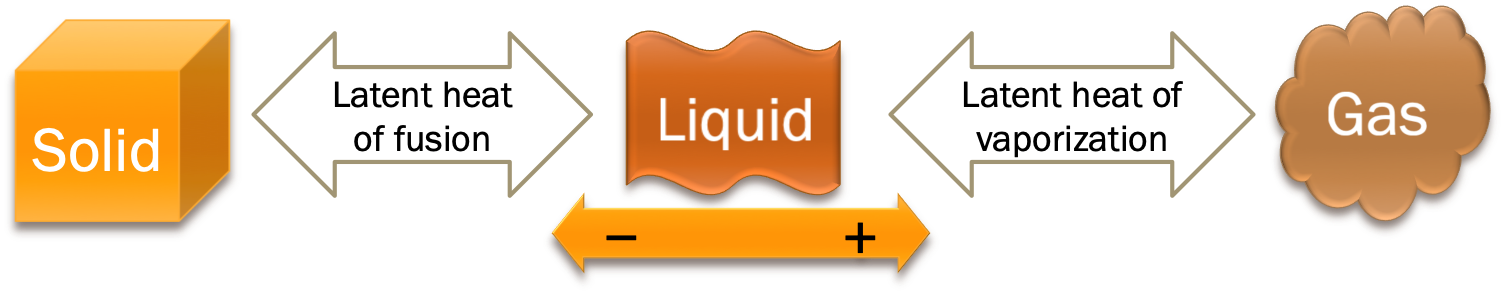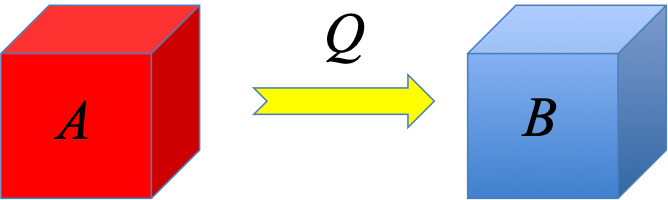# PHYS 170

Lecture 17a - Heat

## Introduction

Heat $Q$ is the transfer of energy due to temperature difference. The symbol for heat is $Q$, SI unit is $J$. A common non-SI unit is $cal$.
Work $W$ accounts for all othe types of energy transfer. The symbol for work is $W$, SI unit is also $J$.

Here is an important convention to keep in mind:

• $Q\gt 0$ when a sample is gaining heat
• $Q\lt 0$ when a sample is losing heat

In the figure one can see the different modes of heat transfer, namely conduction (by contact), convection (by fluid flow), and radiation (by electromagnetic waves).

It may surprise you to know that people used to think heat is totally different from energy (of course scientists had their reasons, which we go into here). As a result, even though science already had a unit for energy ($J$, Joule), scientists used a different unit for heat, $cal$ (calorie).

Definition of calorie ($cal$):

A calorie ($cal$) is the amount of heat required to raise the temperature of $1g$ of liquid water by $1^\circ C$.
The complete definition is actually is a little longer, but this simplified version is close enough for our purpose.

These days, we know that $1cal = 4.186J$, and since heat is a type of energy transfer, $J$ is the SI unit for heat. $cal$ is a common but non-SI unit. We do still use $cal$ in many places, such as the heat content of food labels (which is usually in $Cal = kcal$, "the big cal"), and in calorimetry experiments.

Embed from Getty Images

## Specific Heat Capacity

Specific heat capacity $c$ gives the amount of heat ($Q$) required to raise the temperature of $1kg$ of a sample by $1^\circ C$:

$$Q = mc\Delta T$$
The SI unit of $c$ is $JK^{-1}kg^{-1}$.

Different materials have different values of specific heat capacity.

Specific heat capacity of some common substances (approximate)
Substance $c (JK^{-1}kg^{-1})$
water (liquid) $4186$
steam* $1930$
ice $2090$
copper $385$
gold $129$
porcelain $1100$
salt $900$
*: For a gas the specific heat capacity actually varies greatly depending on the conditions. The value here is $c_p$ at around $100^\circ C$, but we will not get into the details here.

For example, $c$ for liquid water is $4186JK^{-1}kg^{-1}$, meaning if you supply $4186J$ of heat to $1kg$ of liquid water, its temperature would rise by $1K$.

### $T$ vs $\Delta T$ in $^\circ C$ and $K$

$\Delta T_K$ and $\Delta T_C$ always have the same values, as can be seen in the examples below.
Unit Convertion of $T$ and $\Delta T$
$T_i$ $T_f$ $\Delta T_C$ $\Delta T_K$
$0^\circ C = 273 K$ $10^\circ C = 283 K$ $10^\circ C - 0^\circ C = 10^\circ C$ $283K - 273K = 10 K$
$10^\circ C = 283 K$ $30^\circ C = 303 K$ $30^\circ C - 10^\circ C = 20^\circ C$ $303K - 283K = 20 K$
$10^\circ C = 283 K$ $100^\circ C = 373 K$ $100^\circ C - 10^\circ C = 90^\circ C$ $373K - 283K = 90 K$
Note how $\Delta T_K = \Delta T_C$ in all cases, even though $T_K = T_C + 273$. In otherwords, $T$ and $\Delta T$ transform differently under unit conversion.

## Change of Phase and Latent HeatHeat is required during the change of phase, the amount of energy per unit mass is known as the latent heat. The basic equation is given by:

$$Q = \pm m L$$
The $\pm$ is needed because $Q$ is negative when a sample is losing heat (such as when water is being frozen into ice), but positive when it is gaining heat (such as when ice is melting into water). One must remember to use the correct sign when using this equation in computations.

There are two types of latent heat:

Name Symbol Unit Meaning
Latent heat of fusion $L_f$ $J/kg$ heat required to melt $1kg$ of sample at the melting point
Latent heat of vaporization $L_v$ $J/kg$ heat required to vaporize $1kg$ of sample at the boiling point

Latent Heat of Some Common Substances
Substance $L_f (kJ/kg)$ Melting point ($^\circ C$) $L_v (kJ/kg)$ Boiling point ($^\circ C$)
Water 334 0 2254 100
Sulphur 54 115 1406 445
Mercury 11 -39 294 357
Nitrogen 25 -210 199 -196
Oxygen 14 -219 213 -183
Hydrogen 60 -259 449 -253
Note that the unit in the table above is given in $kJ/kg$ instead of $J/kg$ because latent heat tends to be very big numbers in SI unit. In doing calculations, be sure not to forget the "kilo" in the unit or your calculations will all be off by a factor of 1000.

It is also worthwhile comparing the two equations for $Q$ you have encountered so far: $$\left\{ \begin{array}{l} Q = mc\Delta T \text{ [no phase change, \Delta T \neq 0]}\\ Q = \pm mL \text{ [phase change, \Delta T = 0]} \end{array} \right.$$

The absence of $\Delta T$ in the latent heat equation is based on the fact that temperature stays the same during a phase transition (i.e. $\Delta T=0$). For example, as water is boiling, the temperature stays at the boiling point of $100^\circ C$ no matter how strong the flame is.

For a related reason, there is no need to put $\pm$ in the specific heat equation because the sign of $Q$ in $Q=mc\Delta T$ is controlled by the sign of $\Delta T$. For example, when heat is supplied to a sample without phase change, temperature rises ($\Delta T\gt 0$), leading to $Q\gt0$. When it is being cooled, temperature drops ($\Delta T\lt 0$), leading to $Q\lt0$.

## Heat ExchangeSuppose $500J$ of heat has been transferred from $A$ to $B$. Mathematically, this is represented as: $$\begin{eqnarray} Q_A &=& -500J \\ Q_B &=& +500J \end{eqnarray}$$ They clearly satisfy: $$0 = Q_A + Q_B$$ which represents conservation of energy during the heat exchange process.

The above equation can be straight forwardly be generalized to heat exchange amoung multiple objects:

$$0 = Q_A + Q_B + Q_C + \cdots$$

### Try It Yourself (click to show)

Heat $Q$ $J$ energy transfer due to temperature difference
Work $W$ $J$ energy transfer (except heat)
Temperature change $\Delta T$ $K$ difference in temperature, $T_f - T_i$
Specific heat capacity $c$ $JK^{-1}kg^{-1}$ heat to raise $T$ of $1kg$ of sample by $1K$
Latent heat of fusion $L_f$ $Jkg^{-1}$ heat to melt $1kg$ of sample at melting point
Latent heat of vaporization $L_v$ $Jkg^{-1}$ heat to vaporize $1kg$ of sample at boiling point